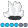Tpham
Quartz | Level 8

## Graphing Yes only by group

I am trying create a graph that shows the row percent only for 2 groups and within the graph show 2-3 variables.. How do I do this?

I created an example in Excel which is attached as an image. I am hoping to do this in SAS, since I have a lot of graphs to create and also want to avoid copying and pasting numbers (so don't want any human error).

I have the raw data, so I will need to use proc freq to compute the percentages.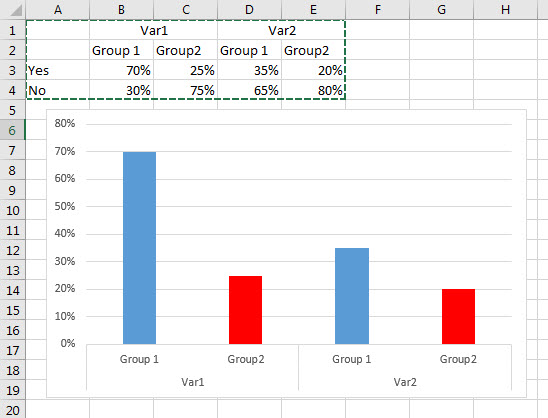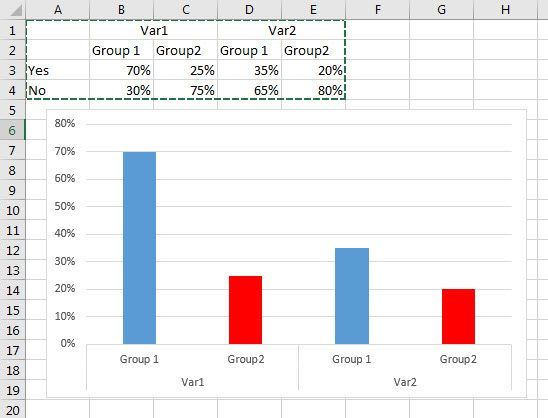1 ACCEPTED SOLUTION

Accepted Solutions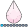djrisks
Barite | Level 11

## Re: Graphing Yes only by group

Okay, here is an example then. I agree with RW9 too, you need to drop the No data before you plot the graph.

data test;
input var\$ group\$ response\$ result;
cards;
var1 group1 yes 0.7
var1 group2 yes 0.75
var1 group1 no 0.3
var1 group2 no 0.25
var2 group1 yes 0.35
var2 group2 yes 0.2
var2 group1 no 0.65
var2 group2 no 0.8
;
run;

proc sgpanel data = test;
where response = "yes";
panelby var;
vbar group / response = result group = response stat= mean;
run;

10 REPLIES 10djrisks
Barite | Level 11

## Re: Graphing Yes only by group

Hi,

What version of SAS do you have please? Can you provide the raw data or the data of the percentages please?

Thanks.Tpham
Quartz | Level 8

## Re: Graphing Yes only by group

SAS 9.2, I can't provide the raw dataset.

I am looking for the general approach I should use so I can code this myself.djrisks
Barite | Level 11

## Re: Graphing Yes only by group

Okay, here is an example then. I agree with RW9 too, you need to drop the No data before you plot the graph.

data test;
input var\$ group\$ response\$ result;
cards;
var1 group1 yes 0.7
var1 group2 yes 0.75
var1 group1 no 0.3
var1 group2 no 0.25
var2 group1 yes 0.35
var2 group2 yes 0.2
var2 group1 no 0.65
var2 group2 no 0.8
;
run;

proc sgpanel data = test;
where response = "yes";
panelby var;
vbar group / response = result group = response stat= mean;
run;

## Re: Graphing Yes only by group

Well, the simplest way would be to have a datastep before it which drops the No data, then its just a barchart by group.

Alternatively, a stacked barchart, with the no group given color white would also work.  You can find many examples of all types of graphs here:

http://blogs.sas.com/content/graphicallyspeaking/?s=stacked

I have filtered for stacked, but there are many examples.Tpham
Quartz | Level 8

## Re: Graphing Yes only by group

If I drop the No data, wouldn't the % be impacted?

Since I want the percentages of "Yes" within the variable for that group...

Is there a way I can make SAS create a bar graph of just numbers? I am thinking if I can use proc freq with an out= option and keep only the percentage of yes within that dataset and graph that?djrisks
Barite | Level 11

## Re: Graphing Yes only by group

You can calculate the percentages using all the data, and then drop the "No data" before you produce the barchart.

## Re: Graphing Yes only by group

So what does your data look like, I assumed from what you had posted you already had percentages, but from the below, you seem to be calculating percentage from data.  Can you clarify what your data looks like, if you already have the percentages then @djrisks solution should work.Tpham
Quartz | Level 8

## Re: Graphing Yes only by group

So my data looks something like this (sample data, not actual). where 1=yes and 2=no and then a group variable 1/2. All these have formats (so var, yes/no and group with group names)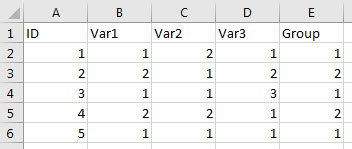So in this case, I want to calculate the row percentage of each variable for each group and then graph only the %yes

The percentage that I have calculated in my excel graph example would be from the proc freq results. I have about 200 vars, of course now 200 variables would be on one graph). So I am wondering is there a way to streamline/automate this?

## Re: Graphing Yes only by group

@Tpham The percents are not impacted because there is only 1 value for each column and if you take the mean or sum of a single number the value is the single number so it graphs the way you expect.

Essentially you're pre-calculating your values and using the above logic to produce your graph.

The example from @djrisks demonstrates this, though you'll need to customize the plot.

## Re: Graphing Yes only by group

Discussion stats
• 10 replies
• 2127 views
• 2 likes
• 4 in conversation